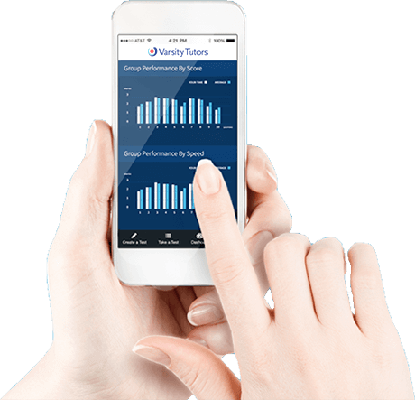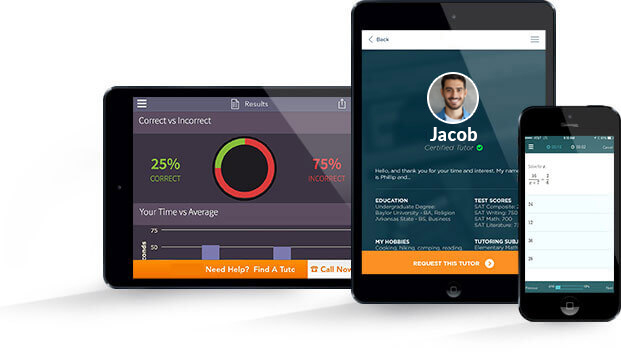# Varsity Tutors Calculus 3 mobile app### The Varsity Tutors Calculus 3 Mobile App

If you are pursuing a physics or other science degree, Calculus 3 is a course you may encounter. It’s not all that different from Calculus 1 and 2, but in this class you’ll apply mathematical concepts in three-dimensions. The math problems you’ll encounter will therefore involve three-dimensional space, multiple integrals, line and surface integrals, and partial derivatives. Mastering the course requires practice and patience; the Varsity Tutors Calculus 3 app enables you to supplement your class work using your iPad, iPhone, or Android device. It is on iTunes and the Google Play Store, and includes all of the available exercises from Varsity Tutor’s Learning Tools.

Full-Length Practice Tests have 20 or 40 questions covering a variety of concepts. The relevant content gives you practice time that can effectively show what you are comfortable with and what concepts you need to put more time and energy into. Each test has a specific difficulty level. At the end, you get immediate feedback, showing your score, how it compares to all other test takers, and your speed on each question. There are also explanations to each answer. These are great study tools as well, helping you focus on your weaknesses and strengthen your skills and confidence in areas you know. You can take the tests as many times as you want and track your progress using the metrics provided. Your results can even be shared with peers, family, teachers, and tutors.

With the practice exercises, you can work on arc length/curvature, cylindrical coordinates, line/plane equations, and spherical coordinates in three-dimensional space. Other topics include minimums and maximums of partial derivatives. There is a review of derivatives and integration, and you’ll be able to practice divergence, vector fields, and other aspects pertaining to line integrals. Double and triple integrals are included as well. With vectors, you’ll be required to work with angles, distances, addition and subtraction, parametric curves, and matrices.

The Full-Length Practice Tests cover these areas and more. You can also narrow it down to individual topics by taking the shorter concept-focused tests on the Calculus 3 app. There are hundreds of these to choose from, so there’s no shortage of practice materials to supplement your class work. Each of the practice tests provides a detailed analysis of your performance, which includes the time it took to take the test as a whole and to answer each individual question, a detailed explanation of the answer, and an analysis of how your performance compared to other test takers. For a more random approach to your studies, you can try the Question of the Day. As you move along, the randomness can test your recollection of complex subjects, as a unique question is selected each day from the catalog of items in all the Calculus 3 practice tests.

Looking for a particular area of focus? The Learn by Concept syllabus lists all the topic areas so you can select exactly what you want to study. For each section and sub-topic, a series of questions and answers is provided, with explanations. You can work on the problem and instantly see how you did. Flashcards are also included. They cover topics question by question, so you can complete as many as you can while gaining valuable insights. Even more, the Flashcards can be completed as they are, or you can add your own text, video, and audio using your smartphone to customize the experience.

These tools are all on the Varsity Tutors Calculus 3 app, which is available now for iOS and Android devices. Get it now to maximize your potential in this course.Calculus 3 is also known as multivariable calculus. In this course, you will progress from working with numbers on a line to working with specific points in space. It allows students to free themselves from the constraints of one-dimension and to use the functions of calculus to describe space, and space to describe the functions. In Calculus 3, you will explore vectors and the geometry of space, vector functions, partial derivatives, multiple integration, and vector calculus. You can study all of these complex topics from any location with the Varsity Tutors Calculus 3 app for iPhones, iPads, and Android devices.

There is no single standardized exam for Calculus 3. Throughout the course, student progress is monitored with quizzes and tests, as well as by reviewing work. The course typically includes a cumulative midterm and final exam on the material covered during the semester. It is very important that you pay close attention to all feedback you receive and use it to create a study plan for the coursework. As with other calculus classes, the information you learn will be necessary to process later coursework. If you do not feel confident in your ability in one area, you should continue to study the principles until you have mastered the concept and are able to confidently move on to the next. One excellent way to do this is with Varsity Tutors’ free Calculus 3 app, available for download from iTunes and the Google Play Store.

Specific points in three-dimensional space can be understood and explored with calculus. The 3D coordinate system will show you how to locate, represent, and draw points and lines within 3D space. It will also allow you to determine the z-axis by utilizing the right-hand rule. You will learn how planes divide space, and the eight octants for the 3D rectangular coordinate system. Vectors, Dot Product, and Cross Product in three-dimensional space will be introduced and explored. Algebraic equations of lines and planes will be reviewed and utilized in writing equations of lines and planes in the 3D coordinate system. Previous courses that laid the foundation of how 2D curves work will help generate 3D curves, known as Quadric Surfaces. You will review polar coordinates and use that knowledge to find the similarities between them and the cylindrical coordinates used in three dimensions.

The topic of vector functions can be broken down to include vector functions and space curves, derivatives and integrals of vector functions, unit tangent vectors, arc length, and curvature. A vector function describes a function whose domain is comprised of real numbers and whose range is comprised of a set of vectors. You will learn how to represent these vector values, find the limit of a vector function, and how to find the domain of vector functions. You will then learn to write and find continuity for a vector function, and how to find and describe a vector function when given parameters to find different space curves.

To understand derivatives and integrals of vector functions, you must first learn to represent the Tangent and Unit Tangent vectors. This will lead to taking higher order derivatives and discussing and identifying smooth curves in space. You will then learn the differentiation formulas for vector functions, and how to find definite and indefinite integrals for vector functions.

The concept of arc length and curvature are covered by reviewing the formulas for parametric, rectangular, and polar equations. Using this knowledge, you will learn to find the length of a curve and how to take the arc length, resolve it for t, and place it back into the original function. This process allows one to identify where they are on a curve once they have been traveling along it.

The Unit Tangent Vector, Unit Normal Vector, and the Binormal Vector will be explored in depth. You will learn the role they play in determining motion in space, and their applications to the motions of spacecraft and differential geometry. You will further learn to calculate these vectors.

The topic of partial derivatives can be further broken down to include functions of variables, limits and continuity, partial derivatives, tangent planes, linear approximations, directional derivatives, and gradient vectors. The functions of variables include the exploration of dependent and independent variables, and the ways in which they impact a function’s range and domain. You will learn to graph the curves of these variables. Building upon the foundational knowledge of limits, you will learn what continuity means by exploring the various types of discontinuity, and learn the two steps needed to prove a function is continuous. The continued study of partial derivatives will allow you to manipulate only one variable at a time while the others remain fixed. This will allow you to see the impact each variable has. The advanced understanding of partial derivatives will allow you to find tangent planes, which then allows one to find linear approximations of a function. Directional derivatives can be found geometrically, and this process will be reviewed. The formula for finding a directional derivative is based on this. The gradient vector is one component of the directional derivative that allows one to find the maximum rate of change of a function, and the direction in which the change is occurring. Finally, you will explore calculus in greater depth with the Multivariable Chain Rule, Relative Extrema, Absolute Extrema, and Lagrange Multipliers. With so many rules to learn, memorize, and apply on command, the comprehensive study tools from Varsity Tutors’ Calculus 3 app for iPhone, iPad, and Android devices are the perfect complement to your coursework.

Multiple integration is primarily concerned with double and triple integrals. When working with double integrals, you will learn to use double integration in polar coordinates, over general regions, with a change of variables, and as they relate to surface area. When working with triple integrals, you will use Cartesian coordinates, cylindrical coordinates, and spherical coordinates.

Vector calculus, which is also known as vector analysis, is a separate branch of mathematics. It is concerned primarily with the differentiation and integration of vector fields, typically within a 3D space. Vector calculus includes the study of vector fields, line integrals, the Fundamental Theorem of Line Integrals, Green’s Theorem, curl and divergence, parametric surfaces and their areas, surface integrals, Stoke’s Theorem, and the Divergence Theorem.

By the end of Calculus 3, you will have a firm understanding of the principles of calculus and how they relate to 3D space. As the concepts of your calculus studies became increasingly more advanced, so did the understanding of the way the world around you works. While it is certainly one of the more challenging courses a student can take, it is well worth the time and effort for anyone who wishes to pursue an advanced degree in the fields of math or science. To get started working to build that future for yourself today, download the Varsity Tutors Calculus 3 app for free from iTunes or the Google Play Store.